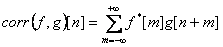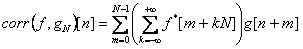Cross-correlation

The following operation is called a discrete cross-correlation of functions f(t) and g(t) defined on Z:The following operation is called a circular discrete cross-correlation of a nonperiodic function f and a periodic function g:Cross-correlation is most often used in signal processing, where f is a pattern, and g is a signal, containing the pattern. A result is a vector of numbers that show how strong the pattern is expressed in the signal.

Data representation

The domain of f and g is Z, but in practice we deal with data with restricted length. The most convenient case is when functions f(t) and g(t) are nonzero only for non-negative t. The ALGLIB package solve this problem: a cross-correlation of two functions, which are nonzero only for non-negative values of the argument. This allows to use a simple relation between the function argument and the array index, where its values are kept: f(t=i) = f_array[i], 0 ≤ i < Mg(t=i) = g_array[i], 0 ≤ i < N. Cross-correlation of these two functions will, however, be nonzero if the argument value is negative.

Most often one is interested in the result of the correlation in positive reports. For example, when processing signals, the correlation of the signal with the pattern at the moment of time t < 0 is almost never important. This is why the ALGLIB package subroutines put the correlation values for the points with positive t to the beginning of the array, while the values for the points with negative t are put to the end. Thus for positive t the index of the array element and t are kept equal.

In case the functions f and g are nonzero both with positive and negative values of the argument, one can utilize the fact that when one of the cross-correlation arguments is shifted, the result is also shifted by the same value (if both arguments are shifted, the result is shifted by the sum of individual shifts). When shifting the signal, the result is shifted in the same direction, when shifting the pattern it is shifted in the reverse direction. Just shift f and g to the right until all the nonzero values are on one side of zero, calculate the cross-correlation and then shift the result back.

Implementation of cross-correlation in ALGLIB

To simplify the program code, ALGLIB implements cross-correlation as a convolution with modified arguments and results. Optimized convolution algorithms are used, which automatically select one of the three different algorithms depending on which of them is faster. The convolution subroutine automatically selects the length of the operands adding zeros when necessary to achieve optimal speed (the speed of FFT used to calculate convolution strongly depends on expanding the length of the operand into prime factors). Thus, ALGLIB users don't have to worry about the optimal operand length.

ALGLIB Project offers you two editions of ALGLIB:

ALGLIB Free Edition:delivered for freeoffers full set of numerical functionalityextensive algorithmic optimizationsno low level optimizationsnon-commercial license

ALGLIB Commercial Edition:flexible pricingoffers full set of numerical functionalityextensive algorithmic optimizationshigh performance (SMP, SIMD)commercial license with support plan

ALGLIB 3.15.0 for C++C++ library.
Delivered with sources.
Monolithic design.
Extreme portability.
Editions:   FREE   COMMERCIAL

ALGLIB 3.15.0 for C#C# library with native kernels.
Delivered with sources.
VB.NET and IronPython wrappers.
Extreme portability.
Editions:   FREE   COMMERCIAL

ALGLIB 3.15.0 for DelphiDelphi wrapper around C core.
Delivered as precompiled binary.
Compatible with FreePascal.
Editions:   FREE   COMMERCIAL

ALGLIB 3.15.0 for CPythonCPython wrapper around C core.
Delivered as precompiled binary.
Editions:   FREE   COMMERCIAL